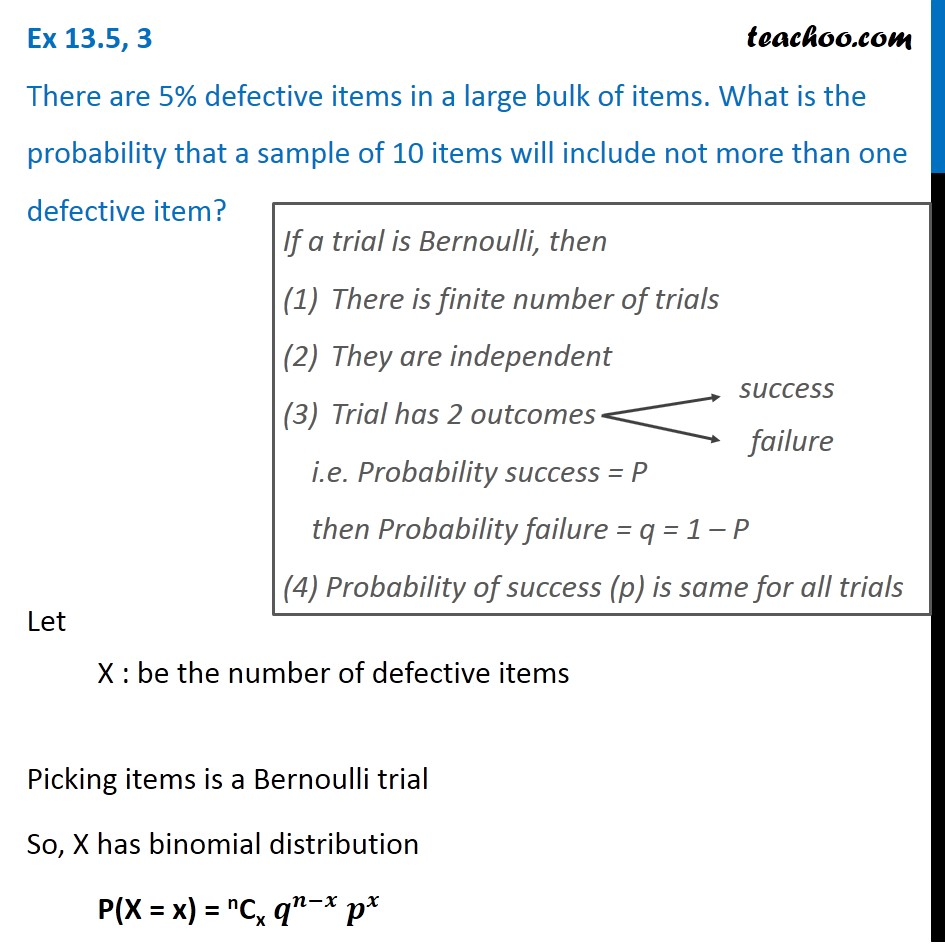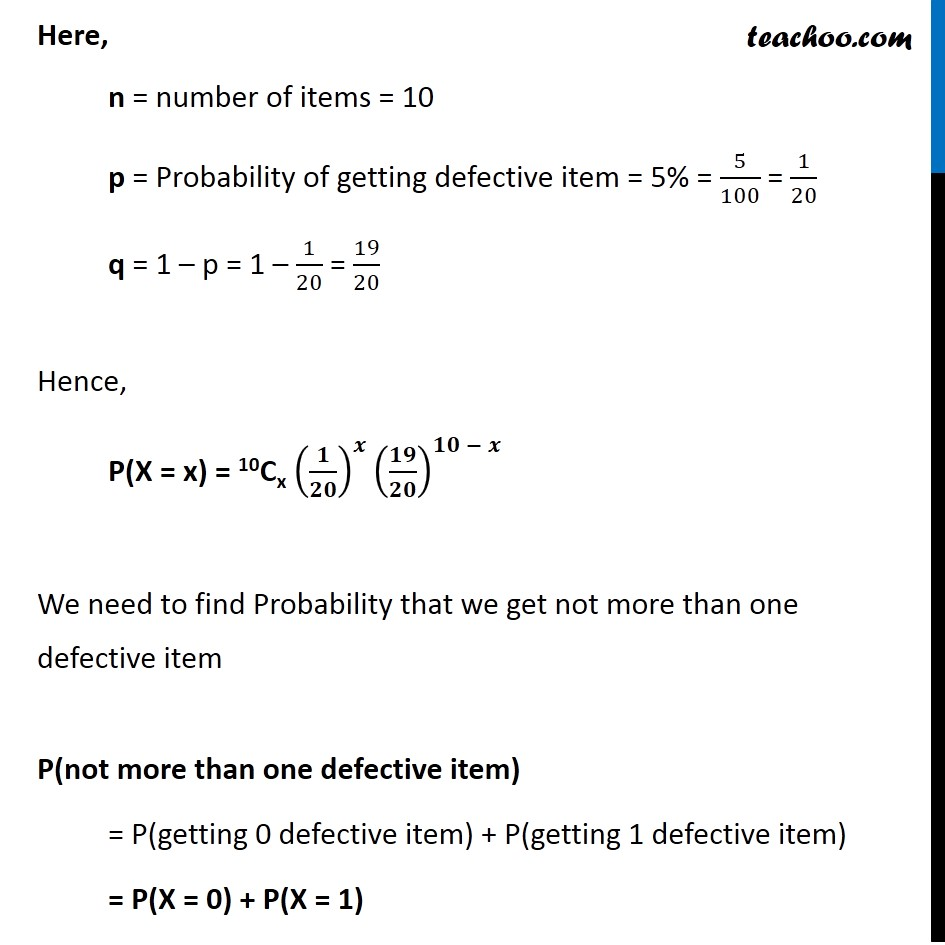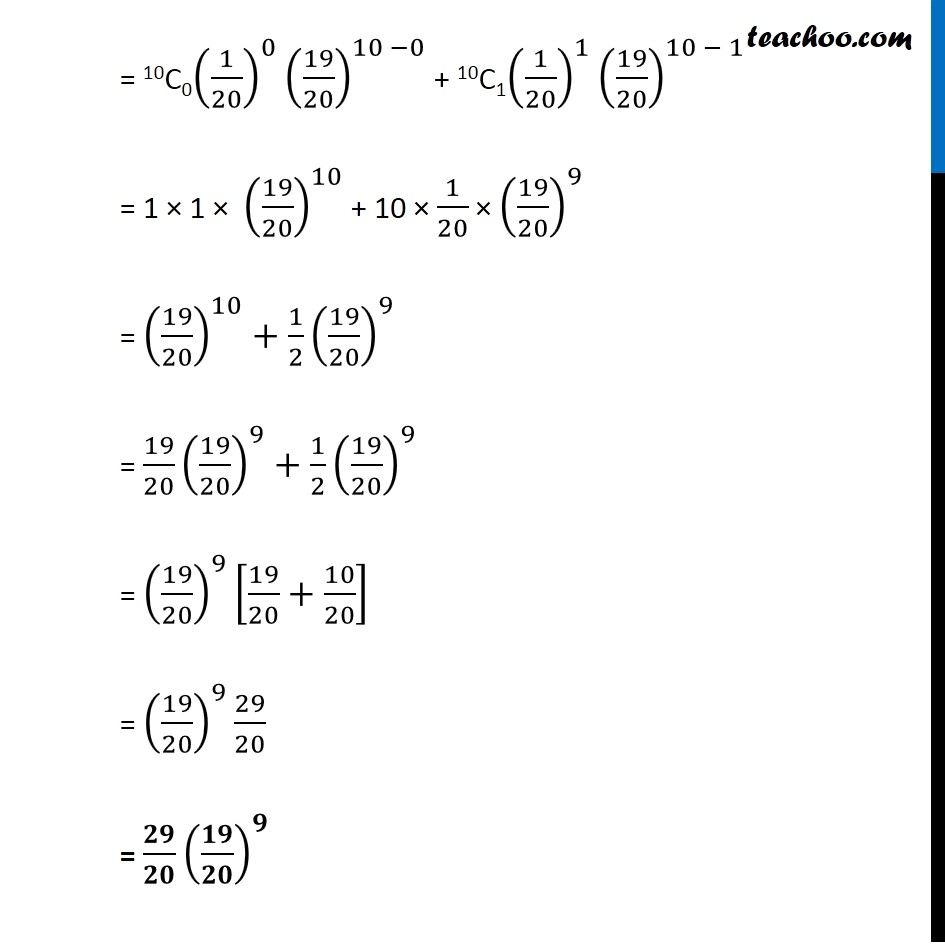Bernoulli Trial

Chapter 13 Class 12 Probability
Serial order wiseLearn in your speed, with individual attention - Teachoo Maths 1-on-1 Class

### Transcript

Question 3 There are 5% defective items in a large bulk of items. What is the probability that a sample of 10 items will include not more than one defective item? If a trial is Bernoulli, then There is finite number of trials They are independenta Trial has 2 outcomes i.e. Probability success = P then Probability failure = q = 1 – P (4) Probability of success (p) is same for all trials Let X : be the number of defective items Picking items is a Bernoulli trial So, X has binomial distribution P(X = x) = nCx 𝒒^(𝒏−𝒙) 𝒑^𝒙 Here, n = number of items = 10 p = Probability of getting defective item = 5% = 5/100 = 1/20 q = 1 – p = 1 – 1/20 = 19/20 Hence, P(X = x) = 10Cx (𝟏/𝟐𝟎)^𝒙 (𝟏𝟗/𝟐𝟎)^(𝟏𝟎 − 𝒙) We need to find Probability that we get not more than one defective item P(not more than one defective item) = P(getting 0 defective item) + P(getting 1 defective item) = P(X = 0) + P(X = 1) = 10C0(1/20)^0 (19/20)^(10 −0) + 10C1(1/20)^1 (19/20)^(10 − 1) = 1 × 1 × (19/20)^10 + 10 × 1/20 × (19/20)^9 = (19/20)^10+1/2 (19/20)^9 = 19/20 (19/20)^9+1/2 (19/20)^9 = (19/20)^9 [19/20+10/20] = (19/20)^9 29/20 = 𝟐𝟗/𝟐𝟎 (𝟏𝟗/𝟐𝟎)^𝟗